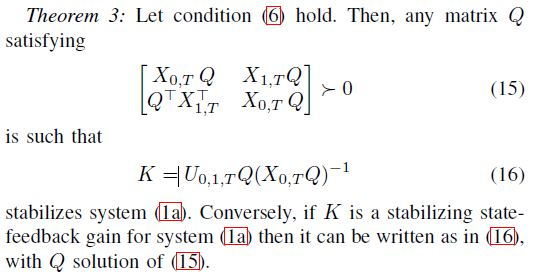# Problem in cvx formHi everyone! I’m new in the use of cvx!
How you can see from the image, I have to solve the condition (15) in order to found the matrix Q. How can I convert this problem in cvx form? (obviuosly , I hava alredy calculated the matrixs X0,t X1,t and U0,1,T).

You have left us in the dark as to what condition (6) is, and how that may or may not bear on the questions below.

CVX does not support strict LMIs (positive definite), only non-strict LMIs (positive semidefinite). So you will need to do something to prevent the matrix of all zeros from being returned for `Q`. Because (15) is homegeneous (any positive multiple of a solution Q of (15) is also a solution of (15), a dehomegenizing constraint, such as perhaps Q + Q^T - I \succeq 0 will need to be added to dehomgenize and prevent the matrix of zeros solution.

I will presume Q is square - if i isn’t, then fix things up. I don’t know the numerical values of `X01`, but if `X01*Q` is not symmetric, you won’t have a proper LMI. Nevertheless, (15) includes Q^T, suggesting Q is not necessarily symmetric, so I won’t declare it so. So you will have to resolve that matter for which sufficient information is not provided in the image for me to properly interpret. With those caveats, here is a rough attempt you may need to fix up.

``````cvx_begin sdp
variable Q(n,n)
[X01*Q  X11*Q; Q'*X11 X01*Q] >= 0
Q + Q' - eye(n) >= 0
cvx_end
``````

As written, I believe CVX will issue a warning about the first LMI not being symmetric (due to the block diagonal terms). You will need to determine how to deal with that. Maybe add a constraint
`X01*Q == Q'*X01'` to make the LMI symmetric?

I recommend you read the entire CVX Users’ Guide, and pay attention to the Semidefinite programming mode chapter http://cvxr.com/cvx/doc/sdp.html .

1 Like

thanks a lot for your help!